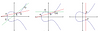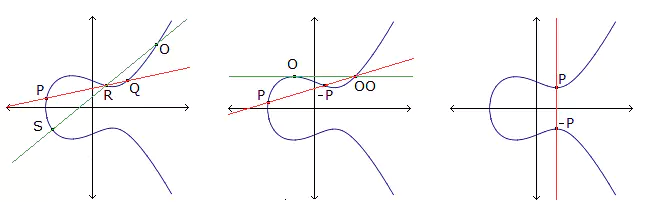# On Complete Arcs Arising from Plane Curves

### Journal article

M. Giulietti, F. Pambianco, F. Torres, E. Ughi
Des. Codes Cryptogr., 2000

Semantic Scholar ArXiv DBLP DOI
Cite

### Cite

APA
Giulietti, M., Pambianco, F., Torres, F., & Ughi, E. (2000). On Complete Arcs Arising from Plane Curves. Des. Codes Cryptogr.

Chicago/Turabian
Giulietti, M., F. Pambianco, F. Torres, and E. Ughi. “On Complete Arcs Arising from Plane Curves.” Des. Codes Cryptogr. (2000).

MLA
Giulietti, M., et al. “On Complete Arcs Arising from Plane Curves.” Des. Codes Cryptogr., 2000.

## Abstract

AbstractWe point out an interplay between $$F_q$$ -Frobenius non-classical plane curves and complete $$\left( {k,d} \right)$$ -arcs in $$P^{\text{2}} \left( {F_q } \right)$$ . A typical example that shows how this works is the one concerning an Hermitian curve. We present some other examples here which give rise to the existence of new complete $$\left( {k,d} \right)$$ -arcs with parameters $$k = d\left( {q - d + 2} \right)$$ and $$d = \left( {q - 1} \right)/\left( {q\prime - 1} \right),q\prime$$ being a power of the characteristic. In addition, for q a square, new complete $$\left( {k,d} \right)$$ -arcs with either $$k = q\sqrt q b + 1$$ and $$d = \left( {\sqrt q + 1} \right)b\left( {2 \leqslant b \leqslant \sqrt q - 1} \right)$$ or $$k = \left( {q - 1} \right)\sqrt q b + \sqrt q + 1$$ and $$d = \left( {\sqrt q + 1} \right)b\left( {2 \leqslant b \leqslant \sqrt q - 2} \right)$$ are constructed by using certain reducible plane curves.

Share